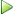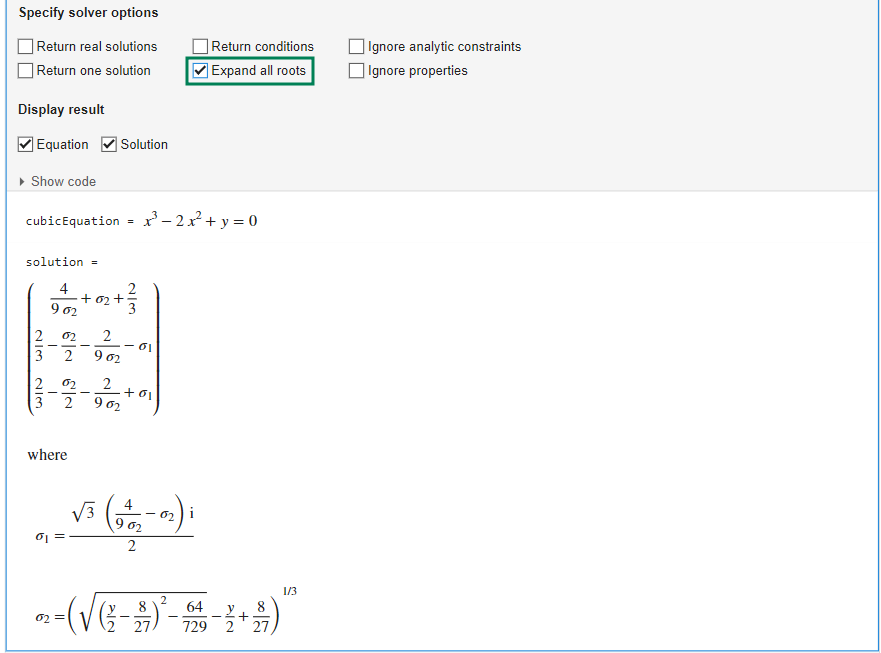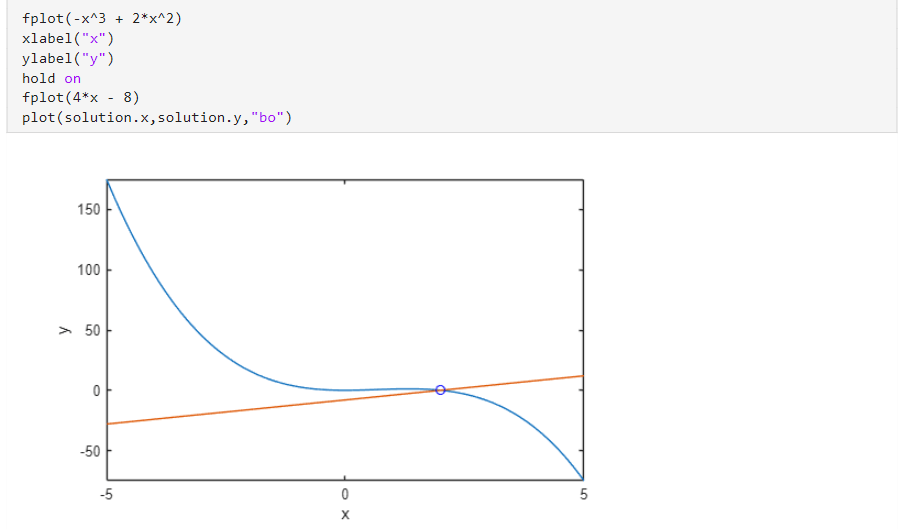# Solve Algebraic Equation Using Live Editor Task

Starting in R2020a, you can interactively solve algebraic equations to obtain symbolic solutions using the Solve Symbolic Equation task in the Live Editor. For more information on Live Editor tasks, see Add Interactive Tasks to a Live Script.

These examples show you how to find the solutions of

• a trigonometric equation

• a cubic equation

• a system of cubic and linear equations

### Solve a Trigonometric Equation

Find the solution of the trigonometric equation $\mathrm{sin}\left(x\right)+\mathrm{cos}\left(x\right)=0$ with the assumption that $x>\pi /2$.

First, go to the Home tab, and create a live script by clickingNew Live Script. Define the symbolic variable `x`, and use the `==` operator to declare the equality sign of the trigonometric equation. Use `assume` to set the assumption on `x`.

```syms x eqn = sin(x) + cos(x) == 0; assume(x > pi/2);```

In the Live Editor tab, run the code by clickingRun to store `x` with its assumption and `eqn` into the current workspace.

Next, open the Solve Symbolic Equation task by selecting Task > Solve Symbolic Equation in the Live Editor tab. To find the solution of the trigonometric equation, select the symbolic equation `eqn` from the workspace. Specify `x` as the variable to solve for. Select the Return conditions option to return the general solution and the analytic constraints under which it holds.You can ignore the assumption on `x` by selecting the Ignore properties option. Return the solution without using the assumption that $x>\pi /2$.To experiment with solving symbolic equations, you can repeat the previous steps for other system equations and solver options. You can run the following examples by adding the code to the existing live script or a new live script.

### Solve a Cubic Equation

Find the solutions of the cubic equation ${\mathit{x}}^{3}-2{\mathit{x}}^{2}+\mathit{y}=0$.

Define the symbolic variables `x` and `y` using `syms`, and use the `==` operator to declare the equality sign of the cubic equation.

```syms x y cubicEquation = x^3 - 2*x^2 + y == 0;```

To find the solutions of the cubic equation, select the symbolic equation `cubicEquation` from the workspace. Specify `x` as the variable to solve for.The solver returns the symbolic solutions in terms of the `root` function. To express the `root` function in terms of square roots, select the Expand all roots option.### Solve a System of Equations

Solve the system of cubic and linear equations:

`$\begin{array}{l}{\mathit{x}}^{3}-2{\mathit{x}}^{2}+\mathit{y}=0\\ \mathit{y}=4\mathit{x}-8\end{array}$`

Define the symbolic variables `x` and `y` using `syms`. Use the `==` operator to declare the equality sign of the equations. To declare the system of equations, combine the two symbolic equations into an array.

```syms x y cubicEquation = x^3 - 2*x^2 + y == 0; linearEquation = y == 4*x - 8; systemEquations = [cubicEquation linearEquation];```

To find the solution of the system of equations, select the symbolic equation `systemEquations` from the workspace. Specify `x` and `y` as the variables to solve for.The solver returns real and complex solutions. To show real solutions only, select the Return real solutions option.### Generate Code

To view the code that a task used, clickat the bottom of the task window. The task displays the code block, which you can cut and paste to use or modify later in the existing script or a different program. For example:Because the underlying code is now part of your live script, you can continue to use the solutions generated by the task for further processing. For example, you can plot the system of equations and their real-valued solution.